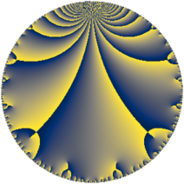# Properties

 Label 45.6.jLevel $45$ Weight $6$ Character orbit 45.j Rep. character $\chi_{45}(4,\cdot)$ Character field $\Q(\zeta_{6})$ Dimension $56$ Newform subspaces $1$ Sturm bound $36$ Trace bound $0$

# Learn more about

## Defining parameters

 Level: $$N$$ $$=$$ $$45 = 3^{2} \cdot 5$$ Weight: $$k$$ $$=$$ $$6$$ Character orbit: $$[\chi]$$ $$=$$ 45.j (of order $$6$$ and degree $$2$$) Character conductor: $$\operatorname{cond}(\chi)$$ $$=$$ $$45$$ Character field: $$\Q(\zeta_{6})$$ Newform subspaces: $$1$$ Sturm bound: $$36$$ Trace bound: $$0$$

## Dimensions

The following table gives the dimensions of various subspaces of $$M_{6}(45, [\chi])$$.

Total New Old
Modular forms 64 64 0
Cusp forms 56 56 0
Eisenstein series 8 8 0

## Trace form

 $$56q + 414q^{4} - 30q^{5} + 12q^{6} + 468q^{9} + O(q^{10})$$ $$56q + 414q^{4} - 30q^{5} + 12q^{6} + 468q^{9} - 68q^{10} - 780q^{11} + 1398q^{14} + 672q^{15} - 5570q^{16} - 8q^{19} + 3522q^{20} - 4848q^{21} - 7554q^{24} - 1654q^{25} + 16968q^{26} + 2700q^{29} + 22578q^{30} - 4436q^{31} - 6268q^{34} - 16344q^{35} + 38016q^{36} + 2508q^{39} + 2036q^{40} - 38928q^{41} - 119400q^{44} - 55890q^{45} + 45764q^{46} + 40244q^{49} - 26466q^{50} - 2964q^{51} + 132894q^{54} + 21024q^{55} - 59442q^{56} + 70200q^{59} + 165084q^{60} + 23368q^{61} - 164668q^{64} - 39210q^{65} + 49452q^{66} - 167076q^{69} + 49752q^{70} + 175176q^{71} + 180168q^{74} - 33528q^{75} - 27348q^{76} - 53480q^{79} + 485892q^{80} - 365112q^{81} - 334242q^{84} + 43936q^{85} - 18060q^{86} - 685536q^{89} - 378936q^{90} - 99576q^{91} + 9614q^{94} - 380028q^{95} - 798q^{96} + 1001340q^{99} + O(q^{100})$$

## Decomposition of $$S_{6}^{\mathrm{new}}(45, [\chi])$$ into newform subspaces

Label Dim. $$A$$ Field CM Traces $q$-expansion
$$a_2$$ $$a_3$$ $$a_5$$ $$a_7$$
45.6.j.a $$56$$ $$7.217$$ None $$0$$ $$0$$ $$-30$$ $$0$$